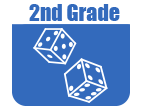IntroductionIn Grade 2, instructional time should focus on four critical areas: (1) extending understanding of base-ten notation; (2) building fluency with addition and subtraction; (3) using standard units of measure; and (4) describing and analyzing shapes.

(1) Students will extend their understanding of the base-ten system. This includes ideas of counting in fives, tens, and multiples of hundreds, tens, and ones, as well as number relationships involving these units, including comparing. Students will understand multi-digit numbers (up to 1,000) written in base-ten notation, recognizing that the digits in each place represent amounts of thousands, hundreds, tens, or ones (for example, 853 is 8 hundreds + 5 tens + 3 ones)

(2) Students will use their understanding of addition to develop fluency with addition and subtraction within 100. They will solve problems within 1,000 by applying their understanding of models for addition and subtraction, and they develop, discuss, and use efficient, accurate, and generalizable methods to compute sums and differences of whole numbers in base-ten notation, using their understanding of place value and the properties of operations. They will select and accurately apply methods that are appropriate for the context and the numbers involved to mentally calculate sums and differences for numbers with only tens or only hundreds.

(3) Students will recognize the need for standard units of measure (such as centimeter and inch) and they use rulers and other measurement tools with the understanding that linear measure involves an iteration of units. They will recognize that the smaller the unit, the more iterations they need to cover a given length.

(4) Students will describe and analyze shapes by examining their sides and angles. Students will investigate, describe, and reason about decomposing and combining shapes to make other shapes. Through building, drawing, and analyzing two- and three-dimensional shapes, students will develop a foundation for understanding area, volume, congruence, similarity, and symmetry in later gradesThe Online Core Resource pages are a collaborative project between the Utah State Board of Education and the Utah Education Network. If you would like to recommend a high quality resource, contact Trish French (Elementary) or Lindsey Henderson (Secondary). If you find inaccuracies or broken links contact resources@uen.org.